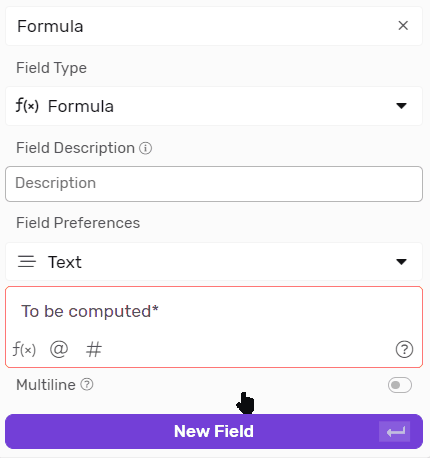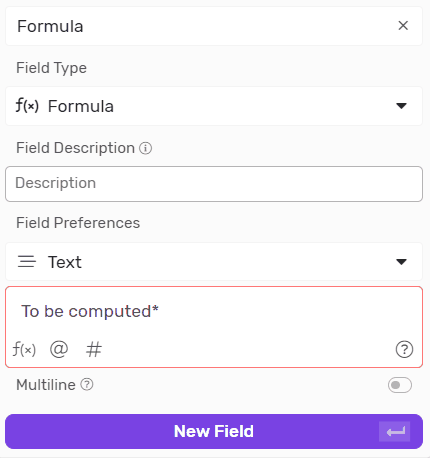# Formula

Formula is a flexible field that gives you the tools you need to shape your data with very high flexibility.Transcript# Creating a Formula field

• Under any list click on fields
• Click + Create New Field
• Type in a field name
• From “Field Type” Drop Down List select “Formula”
• Next you will select the output field type, note that each field type works best with different Functions
• Next you can start building your function# Building the Formula

## Fields

• You can click on the f(x) icon to see the list of fields available under the list you selected in the trigger step.
• You can either scroll to select the field or start typing the field name to narrow down the results.
• E.g. if you want to reference the field named Start Date it will look something like this within the formula {{Start Date}}## Users

• You can click on the @ icon to see the list of users.
• You can either scroll to select the User or start typing the field name to narrow down the results.
• The result of referencing a user should look like this @Mark Kilnger.## Options

• If you’re working with Static List or Status Field you can click on the # icon to see the list of Options.
• You can either scroll to select the Option or start typing the field name to narrow down the results.
• The option or status when referenced should look like this #Qualified#.## Functions

• You can click on the f(x) icon to see the list of Formulas available under the list you selected in the trigger step.
• You can either scroll to select the Formula or start typing the Formula name to narrow down the results.
• E.g. if you want to reference the Formula named Now it will look something like this within the formula Now()# Functions

## Text Functions

Here are a few things you need to know before you start using functions:

• Text is often referred to as a string, since it’s a string of characters (Letters)
• When you’re referring to a field within the formula you need to surround it with double curly brackets, and should look like this {{Field Name}}
• When you’re writing plain text into the formula you need to surround it with double quotes, and should look like this “This is a text”.
• You can reference any field type as a text if you select the output as text.
 Name Function How To Use Examples Result Concatenate Concatenates many strings into a longer one, and can be written in 2 different ways. Concat([Text 1],[Text 2],...,[Text n]) Concat({{First Name}} ,” ” ,”Last Name” ) Jack Klinger Length Count the number of characters in a text. Len([Text]) Len(”Arnold” ) 6 Len({{First Name}} ) *Given that the first name is “Arnold” in this example 6 Left Returns a specific number of characters from the beginning of a string. Left([Text],[Number of characters]) Left(”Arnold”,3 ) Arn Left({{First Name}},3 ) *Given that the first name is “Arnold” in this example Arn Right Returns a specific number of characters from the end of a string. Right([Text] , [Number of characters]) Right(”Arnold”,3 ) Old Right({{First Name}},3 ) *Given that the first name is “Arnold” in this example Old Substring It is the exact opposite of Left() , it Removes the first n characters and returns the rest of the text Substring([text] , [n]) * n is the number of characters to be removed Substring(“ABCDEFG”,2 ) CDEFG Index Of Return the location of String in a bigger String, the first character counts as 0, the second as 1, and so one. Indexof([Search In],[Search For]) IndexOf(“ABCDEFG” , ”CDE” ) 2 * 3rd character return as 2 since we start count from 0 IndexOf(“ABCDEFG” , ”A” ) 0 * 1st Character returns as 0 IndexOf(“ABCDEFG” , ”L” ) -1 * If the String is not found it returns -1 since it has no index Replace To search for a substring and substitute it with another string Replace([Search In],[Search For],[Substitute With]) IndexOf(“ABCDEFG” , ”CD” , ”LM” ) ABLMEFG Trim Removes empty spaces from beginning and end of text. Trim([Text]) Trim(”   Hello There!   ” ) “Hello There!” Trimleft Removes empty spaces from the beginning of text. Trimleft([Text]) Trimleft(”   Hello There!   ” ) “Hello There!   ” Trimright Removes empty spaces from the end of text. Trimright([Text]) Trimright(”   Hello There!   ” ) “   Hello There!” Coalesce Returns the first non null value from a set of parameters Coalesce([Param 1],[Param 2],.....,[Param n]) Format(,,1 ,”Mike” , ,) 1

## Date & Time Functions

 Name Function How To Use Examples Result Now Returns the current Date and Time depending on the timezone of the workspace. Now() - Now UTC Returns the current Date and Time in UTC, i.e GMT+0. NowUTC() * This will only work correctly if the field type is text, if the field type is date it will act like now() - Today Returns the current Datedepending on the timezone of the workspace. Today() - Today UTC Returns the current Date in UTC, i.e GMT+0. TodayUTC() * This will only work correctly if the field type is text, if the field type is date it will act like Today() - Month Return the month from a given date Month([Date]) Month(“2012-12-31” ) 12 Month Name Return the name of the month from the Month’s number MonthName([Number]) MonthName(12 ) December MonthName(Month(“2012-12-31”) ) December Short Month Name Return the short name of the month from the Month’s number ShortMonthName([Number]) ShortMonthName(12 ) Dec ShortMonthName(Month(“2012-12-31”) ) Dec Year Return the year from a given date as a number Year([Date]) Year(“2023-01-22” ) 2023 Format To give a specific format to a given date Format([Date],[Format]) Format(“2023-01-22” ,”yyyy-MMM-dd” ) 2023-Jan-22 Format(“2023-01-22” , ”yyyy.dd.m” ) 2023.22.1 Create Date To create a date from Year, Month and Day CreateDate([Year],[Month],[Day]) CreateDate(2023 ,8 ,23 ) 2023-08-23 Subtract Date Returns the number of days between 2 dates SubtractDate([Date 2],[Date 1]) * Date 2 should be greater than Date 1 otherwise the result will be negative SubtractDate(“2023-08-23” , ”2023-08-18” ) 5 Subtract Time Returns the number of Minutes between 2 dates SubtractTime([Date 2],[Date 1]) * Date 2 should be greater than Date 1 otherwise the result will be negative SubtractTime(“2023-08-23 12:00” , ”2023-08-23  10:00” ) 120 SubtractTime(“2023-08-23 12:00” , ”2023-08-23   10:00” ) / 60 2 * The result was divided by 60 to get the number of hours instead of minutes Get Wroking Days Returns the number of working(business) days between 2 dates GetWorkingDays([Start Date],[End Date],[First Date Of Week (optional)],[Weekend Lenght (optional)]) * End Date should be greater than Start Date otherwise the result will be negative * First Date of Week takes a number between 1 and 7, Default is 1 which is Monday, 7 is Sunday * Weekend Length, is the number of days in the weekend, it's 2 by default GetWorkingDays(“2023-09-25” , ”2023-09-27 ” ) 3

## Mathematical Functions

 Name Description How To Use Examples Result Total Return the total from a set of numbers Sum([Number 1],[Number 2],....,[Number n]) Sum(3 ,4 ,5 ) 12 Average Return the average from a set of numbers Avg([Number 1],[Number 2],....,[Number n]) Avg(3 ,4 ,5 ) 4 Maximum Return the Maximum value from a set of numbers Max([Number 1],[Number 2],....,[Number n]) Max(100 ,33 ,713 ) 713 Minimum Return the Minimum value from a set of numbers Min([Number 1],[Number 2],....,[Number n]) Min(100 ,33 ,713 ) 33 Random Returns a random number between 0 and 1 Rand() 0.4 Random Between Returns a random number between 2 values RandBetween([MIN],[MAX]) RandBetween(10,20) 17 Round Rounds a number to the closest whole, or closest decimal based on the number of digits specified. Round([Number],[Digits after Decimal Point]) Round(3.12847 ) 3 Round(3.12847 ,2 ) 3.13 Round(3.12311 ,2 ) 3.12 Round(3.5 ) 4 Round(3.49 ) 3

## Logical Functions

 Name Description How To Use Examples Result If Evaluates a logical argument, and returns one value if true and another one if false. If([Argument],[Value If True],[Value If False]) If(5 < 7 ,”Tim” ,”Mike” ) Tim If({{Guess}} = 6 ,”Correct” ,”Try Again” ) * Let’s say that guess is 3 in this example Try Again If({{Selling Price}} > {{Cost}} , ”Profit” ,”Loss” ) * Let’s say that Selling Price = 300 and cost = 250 Profit IsBlank Return True in case the parameter has no value assigned to it or it’s an empty string, and false if there’s a value. IsBlank([Param]) IsBlank(”Hi” ) False IsBlank() * No Parameters were presented True IsBlank(”    ” ) * Empty String True IsBlank({{Assignee}} ) * The field is empty in this case and no value is assigned to it. True

# Operators

## Mathematical Operators

 Operator Symbol Sample Result Example Addition + 3 + 5 8 {{Cost}} + {{Shipment Fee}} Subtraction - 9 - 5 4 {{Price}} - {{Cost}} Multiplication * 3 * 7 21 {{Quantity}} * {{Price}} Division / 21 / 7 3 {{Total Price}} / 2 Power ^ 3 ^ 2 9 {{Quantity}} ^ 2 Modulo (Mod) % 9 % 4 1 {{Distance}} % 1000

## Relational Operators

 Operator Symbol Examples Description Equal To = {{Name}} = “John Doe” This will compare whether the value of the field “Name” matches the text “John Doe”. {{Age}} = 13 This will evaluate whether the value of the field “Age” is equal to 13. Not Equal To <> {{Assignee}} <> “Arnold Stognam” This will compare whether the value of the field “Name” matches the text “John Doe”. {{Status}} <> #Blocked# This will evaluate whether the Option in the static field “Status” is not “Blocked”. Greater Than > {{Due Date}} > Today() This will evaluate whether the date in the field “Due Date” is greater than the date of today. Less Than < {{Height}} < 170 This will evaluate whether the value of the field “Height” is less than 170. {{Leave Balance}} < {{Requested Leave Days}} This will evaluate whether the value in the field “Leave Balance” is less than value in the field “Requested Leave Days” Greater Than or Equal to >= {{Price}} >= 3000 This will evaluate whether the value of the field “Price” is greater than or equal to 3000. {{Weight}} >= 100 This will evaluate whether the value of the field “Weight” is greater than or equal to 100. Less Than or Equal to <= {{Quantity}} <= 5 This will evaluate whether the value of the field “Quantity” is less than or equal to 5. {{Score}} <= 50 This will evaluate whether the value of the field “Score” is less than or equal to 50.

## Logical Operators

 Operator Example Description And {{Due Date}} < Today() And {{Status}} <> #Blocked# This will evaluate whether the Due Date is in the past, and the status is not blocked and return true only if both are true, Or {{Ticket Size}} > 5000 Or {{Tier}} = #VIP# This will evaluate whether the ticket size is greater than 5000 or “Tier” is “VIP” and return true if both or either are true.

## Field Type Specific Cases

 Case Description Example Output Getting files name as Text Simply create a formula field and select text as the output field type, then get the file field in the formula builder. Let's say I have a file with the name (January Invoices.xlsx) and I mention the field holding it in the formula builder {{Invoices}} January Invoices.xlsx Getting files count as Number Simply create a formula field and select Number as the output field type, then get the file field in the formula builder. Let's say I have a field called "Attachments" with 7 files in it and I mention the field holding it in the formula builder {{Attachments}} 7### Looking for something else?

Search by entering some keywords such as; 'email automation', 'linked list'...